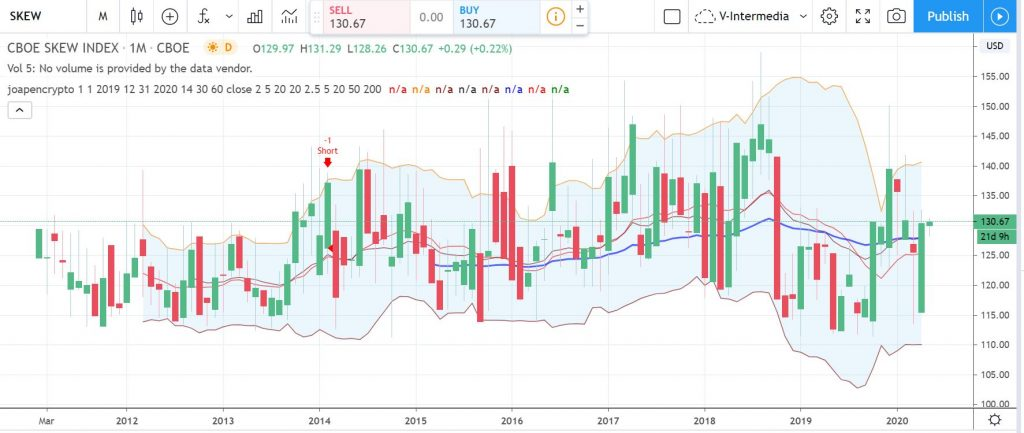# Introduction to SKEW Index

The Cboe SKEW Index (“SKEW”) is an index derived from the price of S&P 500 tail risk. It’s similar to Volatility Index (VIX).

The primary difference between the VIX and the SKEW is that the VIX is based upon implied volatility round the at-the-money (ATM) strike price while the SKEW considers implied volatility of out-of-the-money (OTM) strikes.

SKEW typically ranges from 100 to 150.

• The higher the rating, the higher the perceived tail risk and chance of a black swan event.
• A SKEW rating of 100 means the perceived distribution of S&P 500 returns is normal and, therefore, the probability of an outlier return is small.# Other concepts (for my poor memory)

Out of the Money (OTM) is an expression used to describe an option contract that only contains intrinsic value. These options will have a delta of less than 50.0.

At the money (ATM) is a situation where an option’s strike price is identical to the price of the underlying security.